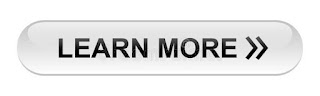222

## \$show=home

### Online Accounting Course  Achieve one of the highest distinctions in the accounting management profession: Become a Online Accountant. As an Online Accountant, you will have the knowledge and the skills to thrive in the competitive accounting industry. The Online Accounting course gives you expertise and real-world skills in accounting analysis. Join more than 100,000 professionals who have been recognized globally for their commitment to ethics and professionalism.## Latest \$type=blogging\$count=10\$author=hide\$comment=hide\$label=hide\$show=home\$date=hide

For determining optimal capital structure, we should learn the importance of optimal capital structure first. Optimal capital structure is that point where our cost of capital and risk of business will be minimum.

So, for determining optimal capital structure, we have to follow following step :

1st Step : Find Different Capital Structure  with Debt Equity Ratio

Capital structure is the mixture of capital and debt. For example, we can take \$100,000 capital and \$ 0 debt or we can take \$ 95000 capital through shares and \$ 5000 debt through issue of debenture. Like this, there may be millions of capital structure by changing the value of share capital and debt value. Total capitalization value will be same. For easy understanding the capital structure, we will calculate debt equity ratio. Debt/equity will be debt equity ratio. For example \$100,000 capital and \$ 0 debt will be 0/100 debt equity. \$ 95000 capital through shares and \$ 5000 debt  will be 5/95 as debt equity ratio. Like this, we can calculate debt equity ratio 10/90, 15/85, 20/80, 25/75, 30/70, 35/65, 40/60, 45/55, 50/50, 55/45, 60/40, 65/35, 70/30, 75/25, 80/20, 85/15 and 90/10

At 90/10, we will at highest risk because we have just \$ 10 capital for repaying loan of \$ 90 and at 10/90, we are at lowest risk because we have \$ 90 capital for repaying \$ 10 loan. But we need to take optimum risk which we reach by taking next steps.

2nd Step : Find the Cost of Capital and Cost of Debt at Different Level of Capital Structure

Cost of capital is dividend rate and cost of debt is interest on loan rate. If there is lowest risk, both cost of capital and cost of debt will low. For example if debt equity ratio is 5/95, it means we have \$ 95 capital for repaying \$ 5 loan, this lowest risky capital structure, so, cost of capital will be 10% and cost of debt will be 4%. But when the risk of repayment of loan will increase due to increasing debt resource and decreasing capital resource, both cost of capital and cost of debt will increase. If debt equity ratio will be 90/10, its is one of highest risky capital structure in which we have just \$ 10 capital for repaying \$ 90 loan, so both capital and debt will become default because it is the basic rule when company will become insolvent, we use all the capital for repaying secured loan. So, \$ 10 capital will become zero. and Rest \$ 80 loan become zero. So, cost of capital will increase upto 16% and cost of debt will increase upto 8%. So, we have to calculate all the cost of capital and cost of debt on different capital structure.

3rd Step : Find the Weighted Average Cost of Capital

If we take the average of cost of debt and cost of capital, it will become average cost of capital. But weighted average cost of capital will give more suitable result. So, we will calculate WACC which is also average cost of capital but we take the product of cost of capital and debt equity ratio. For example, debt equity ratio is 10/90, and cost of capital 10% and cost of debt is 4%, then WACC will be

(0.10 X 4%) + ( 0.90 X 10%) = 9.4%

Like this, you can calculate all the average cost of capital at different level of debt equity ratio and cost of debt and cost of capital level.

4th Step : Reach Optimal Level of Capital Structure  Through Graph

After showing, cost of capital, cost of debt and weighted average cost of capital and debt equity ratio on the graph paper, we can reach optimal level of capital structure. It is the point of debt equity ratio where is the weighted average  cost of capital will be minimum. Following is the graph paper which is taken from Murray State University's website. In simple words, if weighted average cost of capital is 8% and we can make our capital structure by getting loan between \$ 75000 to \$ 70,000 and keeping our capital between \$ 30000 to \$ 25,000.  This will become optimal capital structure.

Related : Meaning of Capital StructureName

ltr
item
Accounting Education: How to Determine Optimal Capital Structure
How to Determine Optimal Capital Structure
http://2.bp.blogspot.com/-KsgCvYiKq7E/UbUiiMLItYI/AAAAAAAALnM/g5F_xOiNTHQ/s640/optimal+capital+structure.PNG
http://2.bp.blogspot.com/-KsgCvYiKq7E/UbUiiMLItYI/AAAAAAAALnM/g5F_xOiNTHQ/s72-c/optimal+capital+structure.PNG
Accounting Education
https://www.svtuition.org/2013/06/how-to-determine-optimal-capital.html
https://www.svtuition.org/
https://www.svtuition.org/
https://www.svtuition.org/2013/06/how-to-determine-optimal-capital.html
true
2410664366776677676
UTF-8